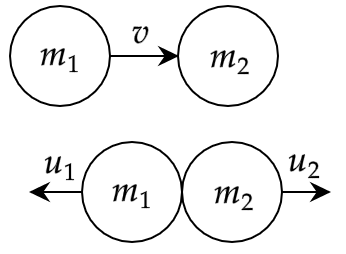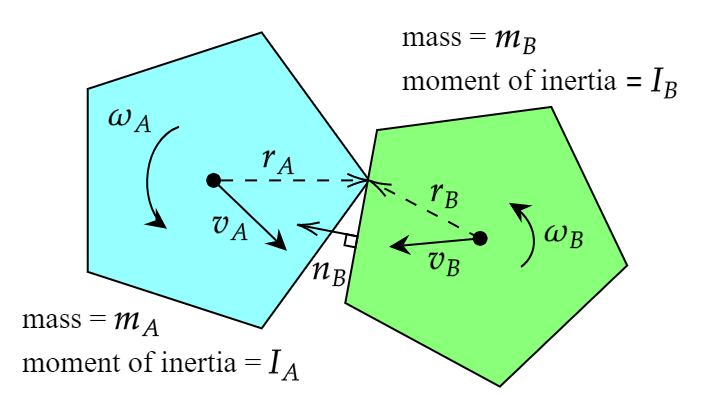# 2D Rigid Body Collision Response

This is a followup to my previous article regarding collision detection between arbitrary polygons. Today I'll be explaining how to update the linear and angular velocity of these polygons in response to that collision. I'm going to go about this with perfectly elastic collision in mind, so both momentum and kinetic energy will be conserved. At the end I'll show how to tweak the formula slightly to change the elasticity with a coefficient of restitution.

There is a great paper here which describes the same collision detection/response algorithm in 3D, and I've used the same notation in case anyone reads both. I found this after getting stuck figuring out how to calculate the magnitude of the impulse exchanged, so thanks to the authors for making that available.

## 1D Collision Response

First I'm going to briefly go over 1D elastic collision response. We have a mass m1 moving at a velocity v colliding with another mass m2. The velocities of m1, m2 after collision will be u1, u2 respectively. We have two unknowns (u1, u2) so we can just combine the conservation equations for momentum and kinetic energy to solve for u1 and u2.$$m_1 v = m_1 u_1 + m_2 u_2$$ $$\frac{1}{2} m_1 v^2 = \frac{1}{2} m_1 u_{1}^2 + \frac{1}{2} m_2 u_{2}^2$$

We end up getting the following values for u1 and u2:

$$u_1 = v - \frac{2 m_2 v}{m_1 + m_2}$$ $$u_2 = \frac{2 m_1 v}{m_1 + m_2}$$

This is a nice result, and it's tempting to try the same method with 2D collision response. But a problem arises because we have 4 equations for conserved quantities: linear momentum on the x-axis, linear momentum on the y-axis, angular momentum, and kinetic energy. But we have 6 unknowns: x-axis velocity, y-axis velocity, and angular velocity for each of two objects post-collision. So we'll have to try a different approach.

## Impulse and Momentum

Suppose we have polygons A and B with masses mA, mB, moments of inertia IA, IB, linear velocity vectors vA, vB, angular velocities ωA, ωB, and vectors from the centers of mass to the point of collision rA, rB. Let a vertex of A be colliding with an edge of B and let vector nB be a unit normal from the colliding edge of B. This is illustrated below.All of these are known values. We get the normal and the point of collision from the previous article on collision detection. I've written another article describing an algorithm for calculating the moment of inertia for an arbitrary polygon, but you can approximate with a simpler formula like for a thin disc depending on what your objects are shaped like.

The key insight from this diagram is that since we're not considering friction, the entire impulse will be applied along nB. This impulse vector, which we'll call J, has units of momentum. An impulse J is applied to A at the point rA, and an equal and opposite impulse -J is applied to B at the point rB. This is related to Newton's second law of motion. We also know how to apply this impulse to compute all the unknowns we need after collision, as shown below. I've used - and + superscripts to denote quantities before and after collision respectively.

$$\vec{J} = j\vec{n_B}$$ $$\vec{v_{A}^{+}} = \vec{v_{A}^{-}} + \frac{1}{m_A} \vec{J} = \vec{v_{A}^{-}} + \frac{j}{m_A} \vec{n_B}$$ $$\vec{v_{B}^{+}} = \vec{v_{B}^{-}} - \frac{1}{m_B} \vec{J} = \vec{v_{B}^{-}} - \frac{j}{m_B} \vec{n_B}$$ $$\omega_{A}^{+} = \omega_{A}^{-} + \frac{\vec{r_A} \times \vec{J}}{I_A} = \omega_{A}^{-} + \frac{j}{I_A} \vec{r_A} \times \vec{n_B}$$ $$\omega_{B}^{+} = \omega_{B}^{-} - \frac{\vec{r_B} \times \vec{J}}{I_B} = \omega_{B}^{-} - \frac{j}{I_B} \vec{r_B} \times \vec{n_B}$$

## Conservation of Energy

If we could only solve for the impulse's magnitude j, we would be able to calculate all the post-collision quantities we need for response! Since we are adding and subtracting the same momentum vector from the system, linear momentum will be conserved no matter what the value of j is. But since this is an elastic collision and we are also conserving kinetic energy, we can use the conservation of kinetic energy to get an equation where j is the only unknown.

$$m_A \|\vec{v_{A}^{-}}\|^2 + I_A {\omega_{A}^{-}}^2 + m_B \|\vec{v_{B}^{-}}\|^2 + I_B {\omega_{B}^{-}}^2 =$$ $$m_A \|\vec{v_{A}^{+}}\|^2 + I_A {\omega_{A}^{+}}^2 + m_B \|\vec{v_{B}^{+}}\|^2 + \frac{1}{2} I_B {\omega_{B}^{+}}^2$$

I've just multipled by 2 on both sides to get rid of some clutter. I haven't expanded any of the post-collision quantities due to the amount of symbols; let's expand and then simplify the first term on the right hand side.

$$m_A \|\vec{v_{A}^{+}}\|^2 = m_A \|\vec{v_{A}^{-}} + \frac{j}{m_A} \vec{n_B}\|^2$$ $$= m_A ((v_{Ax}^{-} + \frac{j}{m_A}n_{Bx})^2 + (v_{Ay}^{-} + \frac{j}{m_A}n_{By})^2)$$ $$= m_A ({v_{Ax}^{-}}^2 + {v_{Ay}^{-}}^2) + m_A (\frac{2j}{m_A} v_{Ax}^{-} n_{Bx} + \frac{2j}{m_A} v_{Ay}^{-} n_{By}) + m_A (\frac{j^2}{m_{A}^2}n_{Bx}^2 + \frac{j^2}{m_{A}^2}n_{By}^2)$$ $$= m_A \|\vec{v_A^{-}}\|^2 + 2j(v_{Ax}^{-} n_{Bx} + v_{Ay}^{-} n_{By}) + \frac{j^2}{m_A} \|\vec{n_B}\|^2$$ $$= m_A \|\vec{v_A^{-}}\|^2 + 2j(\vec{v_A^{-}} \cdot \vec{n_B}) + \frac{j^2}{m_A}$$

We end up with a quadratic equation of j, but notice the first term of the result - it's exactly matched by a term on the left hand side of the conservation of energy equation, so it will cancel out. This ends up happening for all four terms, so the left hand side cancels to zero and we just have the terms with j remaining. When we plug in the other post-collision quantities we get:

$$m_B \|\vec{v_{B}^{+}}\|^2 = m_B \|\vec{v_B^{-}}\|^2 - 2j(\vec{v_B^{-}} \cdot \vec{n_B}) + \frac{j^2}{m_B}$$ $$I_A {\omega_{A}^{+}}^2 = I_A {\omega_{A}^{-}}^2 + 2j\omega_{A}^{-}(\vec{r_A} \times \vec{n_B}) + \frac{j^2}{I_A} (\vec{r_A} \times \vec{n_B})^2$$ $$I_B {\omega_{B}^{+}}^2 = I_B {\omega_{B}^{-}}^2 - 2j\omega_{B}^{-}(\vec{r_A} \times \vec{n_B}) + \frac{j^2}{I_A} (\vec{r_B} \times \vec{n_B})^2$$

Plugging these into the conservation of energy equation and cancelling out all the left hand terms, we get the following:

$$0 = 2j(\vec{v_A^{-}} \cdot \vec{n_B}) + \frac{j^2}{m_A} + 2j\omega_{A}^{-}(\vec{r_A} \times \vec{n_B}) + \frac{j^2}{I_A} (\vec{r_A} \times \vec{n_B})^2$$ $$- 2j(\vec{v_B^{-}} \cdot \vec{n_B}) + \frac{j^2}{m_B} - 2j\omega_{B}^{-}(\vec{r_A} \times \vec{n_B}) + \frac{j^2}{I_A} (\vec{r_B} \times \vec{n_B})^2$$

Since we're not interested in the trivial solution j = 0, we can factor out a j and do some grouping to turn this into a linear function of j (albeit one with quite a few terms).

$$-2(\vec{v_A^{-}} \cdot \vec{n_B} - \vec{v_B^{-}} \cdot \vec{n_B} + \omega_{A}^{-}(\vec{r_A} \times \vec{n_B}) - \omega_{B}^{-}(\vec{r_A} \times \vec{n_B}))$$ $$= j(\frac{1}{m_A} + \frac{1}{m_B} + \frac{(\vec{r_A} \times \vec{n_B})^2}{I_A} + \frac{(\vec{r_B} \times \vec{n_B})^2}{I_B})$$

With this equation we can solve for the only unknown, j, and plug this into our four equations for post-collision linear/angular velocities to solve for those and update our game objects.

## Coefficient of Restitution

It turns out you can change the elasticity of the collision by varying the coefficient of restitution C from 0 (inelastic) to 1 (elastic). You can swap out the -2 coefficient in the above equation with -(1 - C) as described in this paper under section 4.1.

$$(-1 - C)(\vec{v_A^{-}} \cdot \vec{n_B} - \vec{v_B^{-}} \cdot \vec{n_B} + \omega_{A}^{-}(\vec{r_A} \times \vec{n_B}) - \omega_{B}^{-}(\vec{r_A} \times \vec{n_B}))$$ $$= j(\frac{1}{m_A} + \frac{1}{m_B} + \frac{(\vec{r_A} \times \vec{n_B})^2}{I_A} + \frac{(\vec{r_B} \times \vec{n_B})^2}{I_B})$$

## Demo

This demo brings together both collision detection and response. It creates some arbitrary polygons with randomized velocities, sizes, etc and lets them interact for a bit before replacing them with a new set of objects. You can use the slider to change the coefficient of restitution from 0 to 1. There is a heavy rectangular object that is not drawn but bounds the other objects so that they stay interacting longer instead of flying off the screen.

Coefficient of Restitution:

## Limitations

This method works well for two rigid bodies colliding by themselves, but it has some limitations. For one, it doesn't apply any friction forces, which you generally do want for more realism. It also handles objects touching each other at rest rather poorly. More importantly there can still be objects clipping through one another, because when a lighter object is sandwiched between two heavy objects it doesn't transfer force from one heavy object to another, and is often squeezed through one of the heavier objects.

Some problems with objects clipping through one another can be solved by not applying the impulse force unless j > 0. Others require a more complex approach of considering more than two objects at a time.

These problems and others have to be solved by more robust physics engines, but I hope this was a useful introduction to physics of rigid bodies in 2D.#### Robert Fotino

I'm a former computer science student at UCLA, and a game developer in my free time. This site is a place for me to write about whatever I happen to be working on.